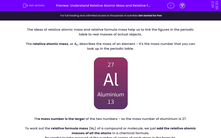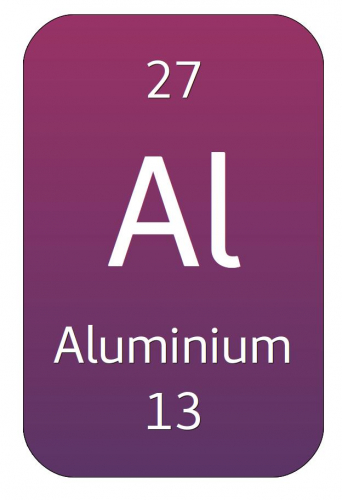# Understand Relative Atomic Mass and Relative Formula Mass

In this worksheet, students will learn the meanings of relative atomic mass and relative formula mass, and how to calculate them.Key stage:  KS 4

Year:  GCSE

GCSE Boards:   AQA, AQA Trilogy, AQA Synergy, OCR 21st Century, OCR Gateway, Pearson Edexcel, Eduqas,

Difficulty level:#### Worksheet Overview

The ideas of relative atomic mass and relative formula mass help us to link the figures in the periodic table to real masses of actual objects.

The relative atomic mass, or Ar, describes the mass of an element - it's the mass number that you can look up in the periodic table.The mass number is the larger of the two numbers - so the mass number of aluminium is 27.

To work out the relative formula mass (Mr) of a compound or molecule, we just add the relative atomic masses of all the atoms in a chemical formula.

Be careful to take account of the number of copies of each atom in the formula.

For example:

What is the relative formula mass of carbon dioxide, CO2?1. Look up the Ar for each of the atoms:   C = 12, O = 16.

2. Add up the relative atomic masses, counting the numbers of each atom. Mr = 12 + (2 x 16) = 44.

It's a good idea to put brackets round the multiplications for each element - this makes sure that you get the right answer.

The reason that relative formula masses are so useful is that they link atomic-scale and real world conservation of mass.

One example of this is when we burn methane:

The word equation is methane + oxygen → carbon dioxide + water.

In symbols, this is CH4 + 2O2 → CO2 + 2H2O

If we look at the molecules, they look like this:The relative formula masses of methane and carbon dioxide are (methane) CH4: 12 + (4 x 1) = 16 and (carbon dioxide) CO2 = 12 + (2 x 16) = 44. The relative formula masses work for any units of mass.

So 16 g methane burns to produce 44 g carbon dioxide. The extra mass comes from the oxygen  needed to make methane burn.

Relative formula masses are a really powerful tool for predicting amounts of chemical produced in reactions. Like any calculations, don't take short cuts, or you will get confused.

Let's move on to some questions.

### What is EdPlace?

We're your National Curriculum aligned online education content provider helping each child succeed in English, maths and science from year 1 to GCSE. With an EdPlace account you’ll be able to track and measure progress, helping each child achieve their best. We build confidence and attainment by personalising each child’s learning at a level that suits them.

Get started